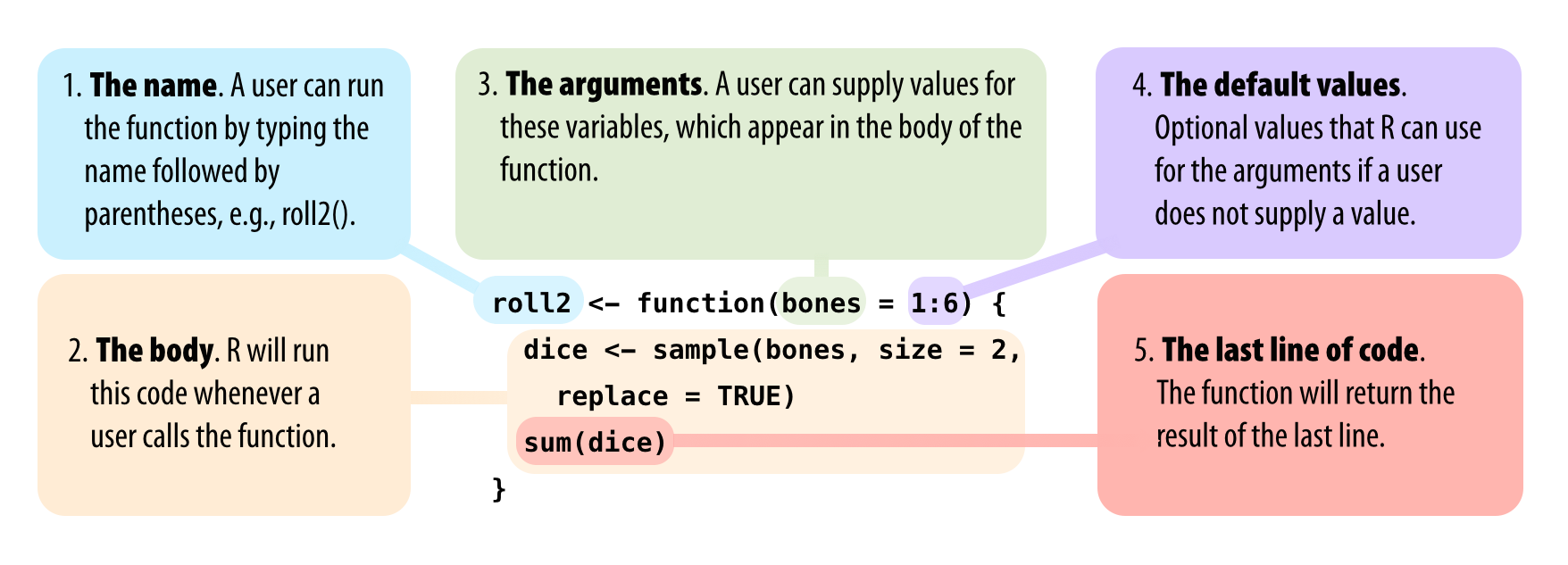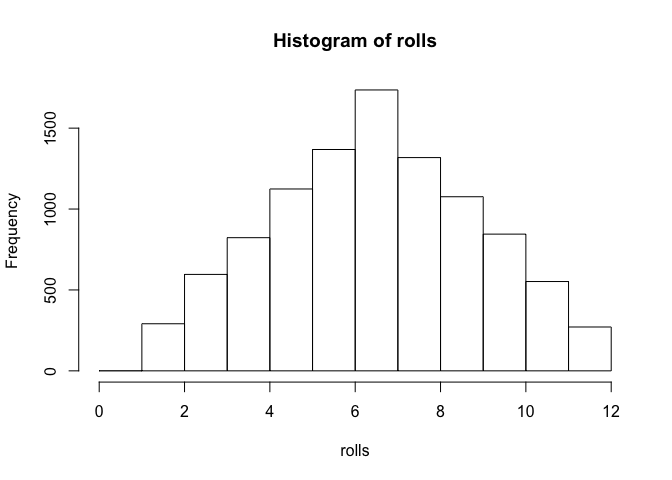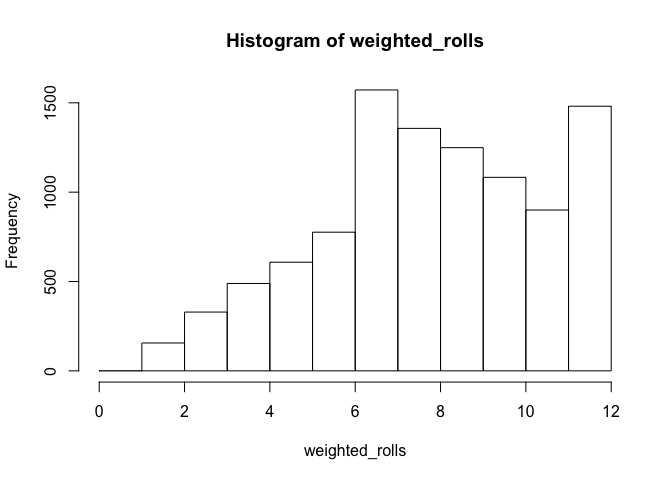# Intro to R Programming

## Today’s Goal

• First mission: assemble R code that will simulate rolling a pair of dice.
• Then, we’ll weight the dice a bit in your favor.

## Roll a Pair of Dice

die <- 1:6
dice <- sample(x = die, size = 2, replace = TRUE)
sum(dice)
##  8
• What is the purpose of the above code?

## Roll a Pair of Dice

die <- 1:6
die
##  1 2 3 4 5 6
dice <- sample(x = die, size = 2, replace = TRUE)
dice
##  6 2
sum(dice)
##  8

## Roll a Pair of Dice

die <- 1:6
dice <- sample(x = die, size = 2, replace = TRUE)
sum(dice)
##  6
die <- 1:6
dice <- sample(x = die, size = 2, replace = TRUE)
sum(dice)
##  8
die <- 1:6
dice <- sample(x = die, size = 2, replace = TRUE)
sum(dice)
##  6

## Roll a Pair of Dice

• What we want to have:
roll()
##  5
roll()
##  6
roll()
##  8

## Writing Your Own Function

• Every function in R has 3 basic parts:
• A name
• A body of code
• A set of arguments
• To create a function in R:
my_function <- function() {}
• function() will build a function out of whatever R code you place between the braces.

## Writing Your Own Function

roll <- function() {
die <- 1:6
dice <- sample(x = die, size = 2, replace = TRUE)
sum(dice)
}
roll()
##  7

## Writing Your Own Function

roll <- function() {
die <- 1:6
dice <- sample(x = die, size = 2, replace = TRUE)
my_sum <- sum(dice)
}
roll()

## Writing Your Own Function

• Code that would display a result:
dice
##  1 5
1 + 2
##  3
sqrt(2)
##  1.414214

## Writing Your Own Function

• Code that would NOT display a result:
dice <- sample(x = die, size = 2, replace = TRUE)
my_sum <- 1 + 2
a <- sqrt(2)

## Function Arguments

roll2 <- function() {
dice <- sample(x = 1:5, size = 2, replace = TRUE)
sum(dice)
}
roll2 <- function() {
dice <- sample(x = 1:10, size = 2, replace = TRUE)
sum(dice)
}

## Function Arguments

roll2 <- function(bones) {
dice <- sample(x = bones, size = 2, replace = TRUE)
sum(dice)
}
roll2(bones = 1:5)
##  2

## Function Arguments

roll2(bones = die)
##  2
roll2(1:4)
##  3
roll2()
## Error in sample(x = bones, size = 2, replace = TRUE): argument "bones" is missing, with no default

# Default Value in Function Arguments

roll2 <- function(bones = 1:6) {
dice <- sample(x = bones, size = 2, replace = TRUE)
sum(dice)
}
roll2()
##  8
roll2(bones = 1:5)
##  7

## Function Arguments## Today’s Goals

• First mission: assemble R code that will simulate rolling a pair of dice.
roll2 <- function(bones = 1:6) {
dice <- sample(x = bones, size = 2, replace = TRUE)
sum(dice)
}

## Getting Help in R

?sample

Let’s switch over to RStudio!

## Sampling with Weights

weighted_roll <- function() {
die <- 1:6
dice <- sample(x = die, size = 2, replace = TRUE,
prob = c(1/8, 1/8, 1/8, 1/8, 1/8, 3/8))
sum(dice)
}
weighted_roll()
##  9
weighted_roll()
##  12

## Is It Working?

rolls <- replicate(n = 10000, expr = roll())
hist(x = rolls, breaks = c(0:12))## Is It Working?

weighted_rolls <- replicate(n = 10000, expr = weighted_roll())
hist(x = weighted_rolls, breaks = c(0:12))